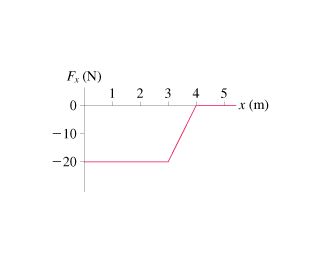# Problem: A 100 g particle experiences the one-dimensional, conservative force Fx shown in the figure.Let the zero of the potential energy be at x = 0 m.What is the potential energy at x = 1.0, 2.0, 3.0, and 4.0 m? Hint: Think about the definition of potential energy and the geometric interpretation of the work done by a varying force.Suppose the particle is shot toward the right from x = 1.0 m with a speed of 24 m/s. Where is the particles turning point?

###### FREE Expert Solution
81% (472 ratings)
###### Problem Details

A 100 g particle experiences the one-dimensional, conservative force Fx shown in the figure.

Let the zero of the potential energy be at x = 0 m.What is the potential energy at x = 1.0, 2.0, 3.0, and 4.0 m? Hint: Think about the definition of potential energy and the geometric interpretation of the work done by a varying force.

Suppose the particle is shot toward the right from x = 1.0 m with a speed of 24 m/s. Where is the particles turning point?

Frequently Asked Questions

What scientific concept do you need to know in order to solve this problem?

Our tutors have indicated that to solve this problem you will need to apply the Force & Potential Energy concept. You can view video lessons to learn Force & Potential Energy. Or if you need more Force & Potential Energy practice, you can also practice Force & Potential Energy practice problems.